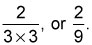##### ASVAB AFQT For Dummies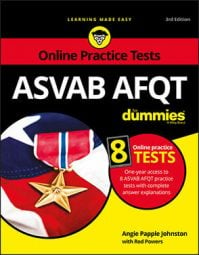Simplifying (or reducing) fractions means to make the fraction as simple as possible. You're usually required to simplify fractions on the ASVAB AFQT math subtests before you can select the correct answer.

For example, if you worked out a problem and the answer was 4/8, the correct answer choice on the math subtest would probably be 1/2, which is the simplest equivalent to 4/8.

Many methods of simplifying fractions are available. Following are two of the easiest methods; you can decide which is best for you.

## Method 1: Divide by the lowest prime numbers

Try dividing the numerator and denominator by the lowest prime numbers until you can't go any further.

For example, simplify 24/108.

Both the numerator and denominator are even numbers, so they can be divided by the lowest prime number, which is 2.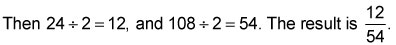The numerator and denominator are both still even numbers, so divide by 2 again: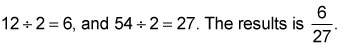This time the denominator is an odd number, so you know it isn't divisible by 2. Try the next highest prime number, which is 3: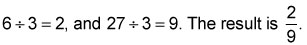Because no common prime numbers divide evenly into both 2 and 9, the fraction is fully simplified.

## Method 2: List prime factors

With this method, you simply list the prime factors of both the numerator and the denominator, and then see whether any cancel out (are the same).

Once again, simplify 24/108.

The prime factors of 24 are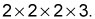The prime factors of 108 are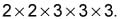You can now write the fraction as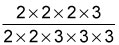Two of the 2s and one of the 3s cancel out, so you can remove them from both the numerator and the denominator. What's left is